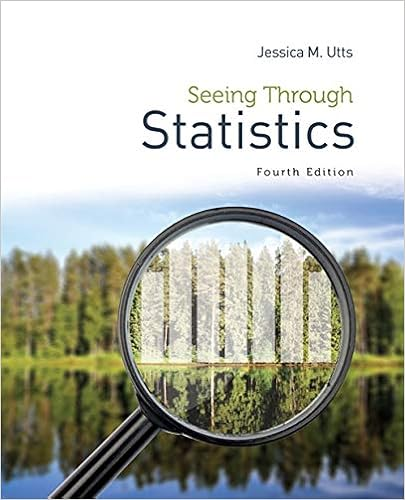# If the procedure is repeated many times we would

• Homework Help
• 30
• 100% (5) 5 out of 5 people found this document helpful

This preview shows page 7 - 11 out of 30 pages.

##### We have textbook solutions for you!
The document you are viewing contains questions related to this textbook.The document you are viewing contains questions related to this textbook.
Chapter 22 / Exercise 2
Seeing Through Statistics
UttsExpert Verified
to their designated wake-up time to fall in 90% of the resulting 90% confidence intervals.If the procedure is repeated many times, we would expect about 90% of the resulting confidence intervals to contain thepopulation mean adrenocorticotropin level in people one hour prior to their designated wake-up time.If the procedure is repeated many times, then we’d expect the population mean adrenocorticotropin level in people one hourprior to their designated wake-up time to fall in 90% of the resulting 90% confidence intervals.Answer Key:3.eIf the same data were used to create a 95% confidence interval to estimate the population mean adrenocorticotropin level in people onehour prior to their designated wake-up time, the resulting interval would be:wider than the 90% interval.
##### We have textbook solutions for you!
The document you are viewing contains questions related to this textbook.The document you are viewing contains questions related to this textbook.
Chapter 22 / Exercise 2
Seeing Through Statistics
UttsExpert Verified
narrower than the 90% interval.the same width as the 90% interval.wider than the 90% interval.Answer Key:Explanation:With a 95% confidence level, the multiplier t* will be larger as compared ot the multiplier for a 90% confidence interval.
Return to the topGo to question:Question 4 Background :Caffiene Content in ColasYou want to estimate the mean caffeine content per 12-ounce bottle of a cola drink. For your study, a random sample of 12-ounce colabottles was collected. A 95% confidence interval for the population mean caffeine content per 12-ounce bottle is found to be 36.88milligrams to 41.52 milligrams.Question 4 Subquestions12356789104
1/point1 4.aFind the estimate for the mean caffeine content in a 12-ounce cola bottle. Include units.Student Answer:
1/pointA fixed level of significance of 5% was selected to test the hypotheses H: μ = 40 milligrams versus the alternative H: µ ≠ 40 milligrams.What would be your statistical decision?areject the null hypothesisfail to reject the null hypothesiscannot tellfail to reject the null hypothesisAnswer Key:Explanation:Because the value 40 is in the 95% confidence interval, it is a reasonable value for the population mean caffeine content in 12-ounce cola bottles. Thus, we would fail to reject Hat a 5% significance level.
1/pointA fixed level of significance of 10% was selected to test the hypotheses H: µ = 37 milligrams versus the alternative H: µ ≠ 37milligrams. What would be your statistical decision?
4.bA fixed level of significance of 5% was selected to test the hypotheses H: μ = 40 milligrams versus the alternative H: µ ≠ 40 milligrams.What would be your statistical decision?0areject the null hypothesisfail to reject the null hypothesiscannot tellfail to reject the null hypothesisAnswer Key:Explanation:Because the value 40 is in the 95% confidence interval, it is a reasonable value for the population mean caffeine content in 12-ounce cola bottles. Thus, we would fail to reject Hat a 5% significance level.
04.cA fixed level of significance of 10% was selected to test the hypotheses H: µ = 37 milligrams versus the alternative H: µ ≠ 37milligrams. What would be your statistical decision?
•••